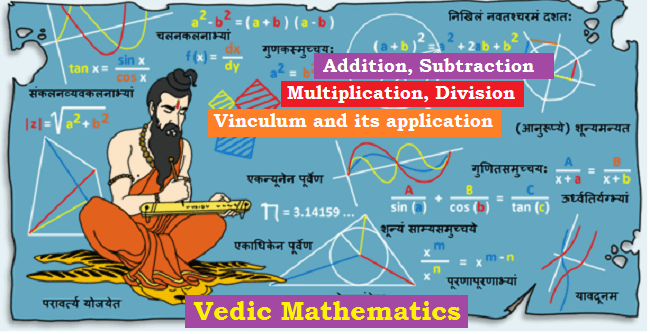# (Vedic Mathematics) Addition, Subtraction, Multiplication, Division and VinculumVedic Sutras

Increasing (एकाधिक) a number to the digit which comes before (पूर्व)

It is represented by a dot on the number which has to be increased.

2. Ek Nyunen Purven (एक न्यूनेन पूर्वेण):

Decreasing (एक न्यूनेन) a number to the digit which comes before (पूर्व)

Put a dot (nyun) below the unit place of the number to find ek nyunen of that number.

For example, ek nyunen of 57 = 57-1 = 56

3. Vinculum (विनकूलम) Number:

Negative numbers are called VInculum and are represented by a bar on the number.e.g. 2 (called Vinculum 2)= -2

For simplifying calculations 10 aur multiples of 10 is often taken as Adhar (Base).

Upadhar (Sub-base) is a multiple of Base. For example, if Base is 10, 10x is a Sub-base.

Vichalan (Deviation) is deviation from the Base.

Vichalan (Deviation) = Number – Base

5. Nikhilam – navatah charanam dashatah (निखिलमनवतः चरणम दशतः):

In ancient Indian mathematician, number 9 was called as Param or Brahma Number (परम या ब्रह्मा अंक) and number 10 was called as Purn Number (पूर्ण अंक).

Nikhilam means- Charam (unit digit) from 10 and others from 9

For example; it can be used for converting a number in to its Vinculum Number.

6. Urdharva Triyagyabhyam (उर्ध्व तिर्यग्भयाम):

Urdharva: Above or straight (ऊपर या सीधा)

Tiryak: Cross (तिरछा)

7. Paravartya Yojyet (परावर्त्य योजयेत):

Paravartya Yojyet means use after changing signs.

(+) becomes (-), (-) becomes (+), (x) becomes (÷) and (÷) becomes (x)

Let’s take an example, 549 + 234 + 403

Subtraction

Find 34 – 18

2. Ek Nyunen Purven (एक न्यूनेन पूर्वेण):

Find 560-374

Let’s take an example: 423-654+847-126+204

Our example may be represented as (using Vinculum)

• Rekhank digit can be subtracted from 10 which affect the digit left to it.
• 1 will become (10-1)= 9
• 7 will become (7-1)= 6
• Therefore final answer is 6/9/4= 694

Multiplication

Multiplication of two numbers by this method is quite easy when the sum of their Charam Numbers (Units Digits) is 10 or powers of 10.

Let’s take an example: 95 x 95 (Charam Numbers 5+5=10)

Let’s take another example, 586 x 514 (86+14=100)

2. Ek Nyunen Purven (एक न्यूनेन पूर्वेण):

Take an example, 8 x 9

Take another example, 68 x 999

Let’s take an example, 12 x 14 (Base=10)

Let’s take another example, 92 x 87 (Base=100)

There will be only 2 digits in the right part (as Base=100), therefore the product 104 is taken as 04 and 1 is carried over to the left part.

Division

Take an example: 311÷8 (Base=10)

Take another example, 10025 ÷88

2. Urdharva Triyagyabhyam (उर्ध्व तिर्यग्भयाम):

Take an example, 23754 ÷74

3. Paravartya Yojyet (परावर्त्य योजयेत):

Take an example, 1358 ÷ 113 (Base=100)